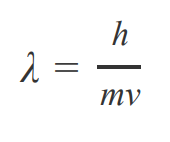# Problem: The mass of a golf ball is 45.9 g. If it leaves the tee with a speed of 68.0 m/s , what is its corresponding wavelength?Just as light waves have particle behavior, a moving particle has a wave nature. The faster the particle is moving, the higher its kinetic energy and the shorter its wavelength. The wavelength, λ, of a particle of mass mm, and moving at velocity v, is given by the de Broglie relationwhere h = 6.626 × 10− 34 J ⋅ s is Planck's constant.This formula applies to all objects, regardless of size, but the de Broglie wavelength of macro objects is miniscule compared to their size, so we cannot observe their wave properties. In contrast, the wave properties of subatomic particles can be seen in such experiments as diffraction of electrons by a metal crystal.

🤓 Based on our data, we think this question is relevant for Professor Davis & Chen's class at McMaster University.

###### FREE Expert SolutionView Complete Written Solution
###### Problem Details

The mass of a golf ball is 45.9 g. If it leaves the tee with a speed of 68.0 m/s , what is its corresponding wavelength?

Just as light waves have particle behavior, a moving particle has a wave nature. The faster the particle is moving, the higher its kinetic energy and the shorter its wavelength. The wavelength, λ, of a particle of mass mm, and moving at velocity v, is given by the de Broglie relationwhere h = 6.626 × 10− 34 J ⋅ s is Planck's constant.

This formula applies to all objects, regardless of size, but the de Broglie wavelength of macro objects is miniscule compared to their size, so we cannot observe their wave properties. In contrast, the wave properties of subatomic particles can be seen in such experiments as diffraction of electrons by a metal crystal.